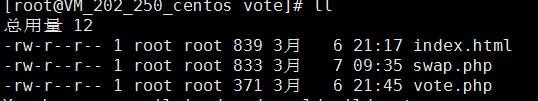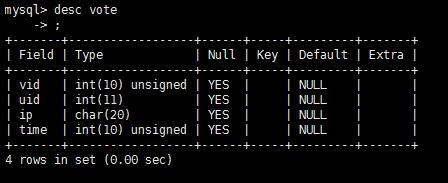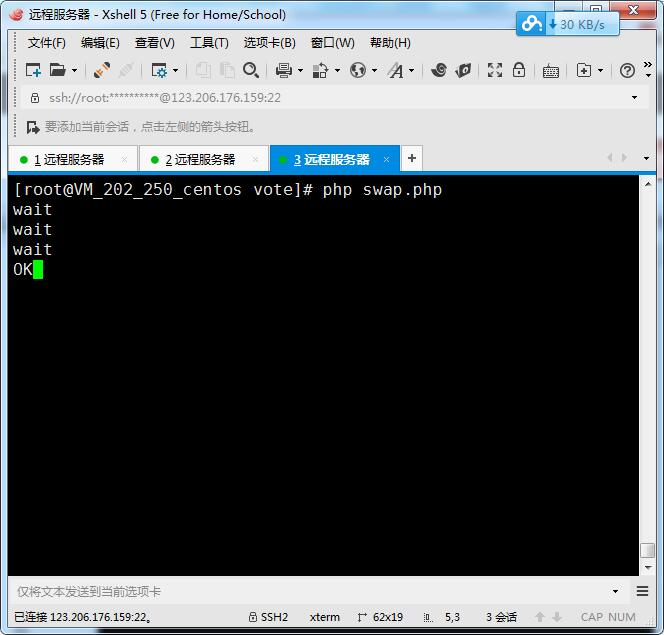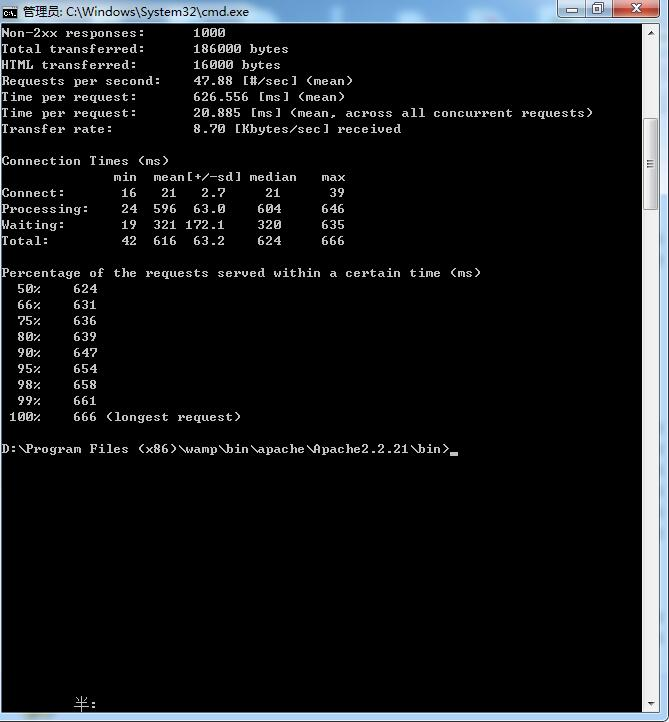# Redis+MySQL冷热数据交换index.html文件

<!DOCTYPE html PUBLIC "-//W3C//DTD XHTML 1.0 Strict//EN" "http://www.w3.org/TR/xhtml1/DTD/xhtml1-strict.dtd">
<html xmlns="http://www.w3.org/1999/xhtml" xml:lang="en">
<meta http-equiv="Content-Type" content="text/html;charset=UTF-8">
<title>Document</title>
<script src="http://lib.sinaapp.com/js/jquery/1.9.1/jquery-1.9.1.min.js"></script>
<body>
<p><span id="uid1">0</span><input type="button" value="用户1" onclick="vote(1);" /></p>
<p><span id="uid2">0</span><input type="button" value="用户2" onclick="vote(2);" /></p>
<p><span id="uid3">0</span><input type="button" value="用户3" onclick="vote(3);" /></p>
</body>
<script>
function vote(i){
$.get('vote.php?uid='+i,function(rs){ var span = '#uid'+i;$(span).html(rs);
});
}
</script>
</html>


vote.php

<?php
$redis = new Redis();$redis->connect('localhost',6379);
//计算每个用户的总票数
$uid = intval($_GET['uid']);
//$uid = mt_rand(1,3);//随机指定投票人员，方便进行压力测试 echo$redis->incr($uid);$voteid = $redis->incr('global_voteid');$redis->set('vote:'.$voteid.':uid',$uid);
$ip =$_SERVER['REMOTE_ADDR'];
$redis->set('vote:'.$voteid.':ip',$ip);$redis->set('vote:'.$voteid.':time',time());  重点内容 这个文件主要实现冷热数据交换，首先连接MySQL数据库和redis服务器，然后每隔5秒去执行while循环，在while循环里获取自增长的投票主键和最近一次插入mysql的投票主键（位置）。判断插入的位置是否存在，如果不存在就从头插入，如果全部插入完毕，就进行等待，如果没有插入完毕，就进行插入操作。 <?php //连接数据库$pdo = new PDO('mysql:host=localhost;dbname=test','root','1234');
$pdo->query('set names utf8'); //连接redis$redis = new Redis();
$redis->connect('localhost',6379); //永真循环 while(true){$vid = $redis->get('global_voteid');//自增长的主键$last = $redis->get('last');//最近一次插入mysql的投票主键 //如果没有插入数据库，刚开始的肯定为true if(!$last){
$last = 0;//设置为0 } //如果所有的数据都被插入到MySQL中 if($vid == $last){ echo "wait\n";//输出等待 }else{ //进行插入到数据库操作$sql = 'insert into vote(vid,uid,ip,time) values';
for($i =$vid;$i>$last;$i--){$k1 = 'vote:'.$i.':uid';$k2 = 'vote:'.$i.':ip';$k3 = 'vote:'.$i.':time';$row = $redis->mget([$k1,$k2,$k3]);
$sql.="($i,$row,'$row',$row),";$redis->delete($k1,$k2,$k3); }$sql = substr($sql,0,-1);$pdo->exec($sql);$redis->set('last',\$vid);//设置插入的主键位置
echo 'OK';
}
sleep(5);//每隔5秒执行循环
}


vote表1、使用php命令行工具调用swap.php2、使用Apache的ab工具进行压力测试。©️2019 CSDN 皮肤主题: 像素格子 设计师: CSDN官方博客